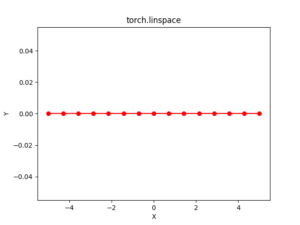# Python Pytorch linspace() method

PyTorch is an open-source machine learning library developed by Facebook. It is used for deep neural network and natural language processing purposes.

The function `torch.linspace()` returns a one-dimensional tensor of steps equally spaced points between start and end.

The output tensor is 1-D of size steps.

Syntax: torch.linspace(start, end, steps=100, out=None)

Parameters:
start: the starting value for the set of point.
end: the ending value for the set of points
steps: the gap between each pair of adjacent points. Default: 100.
out(Tensor, optional): the output tensor

Return type: A tensor

Code #1:

 `# Importing the PyTorch library ` `import` `torch ` ` `  `# Applying the linspace function and ` `# storing the resulting tensor in 't' ` `a ``=` `torch.linspace(``3``, ``10``, ``5``) ` `print``(``"a = "``, a) ` ` `  `b ``=` `torch.linspace(start ``=``-``10``, end ``=` `10``, steps ``=` `5``) ` `print``(``"b = "``, b) `

Output:

```a =  tensor([ 3.0000,  4.7500,  6.5000,  8.2500, 10.0000])
b =  tensor([-10.,  -5.,   0.,   5.,  10.])
```

Code #2: Visualization

 `# Importing the PyTorch library ` `import` `torch ` `# Importing the NumPy library ` `import` `numpy as np ` ` `  `# Importing the matplotlib.pylot function ` `import` `matplotlib.pyplot as plt ` ` `  `# Applying the linspace function to get a tensor of size 15 with values from -5 to 5 ` `a ``=` `torch.linspace(``-``5``, ``5``, ``15``) ` `print``(a) ` ` `  `# Plotting ` `plt.plot(a.numpy(), np.zeros(a.numpy().shape), color ``=` `'red'``, marker ``=` `"o"``)  ` `plt.title(``"torch.linspace"``)  ` `plt.xlabel(``"X"``)  ` `plt.ylabel(``"Y"``)  ` ` `  `plt.show() `

Output:

```tensor([-5.0000, -4.2857, -3.5714, -2.8571, -2.1429, -1.4286, -0.7143,  0.0000,
0.7143,  1.4286,  2.1429,  2.8571,  3.5714,  4.2857,  5.0000])

[torch.FloatTensor of size 15]
```My Personal Notes arrow_drop_upCheck out this Author's contributed articles.

If you like GeeksforGeeks and would like to contribute, you can also write an article using contribute.geeksforgeeks.org or mail your article to contribute@geeksforgeeks.org. See your article appearing on the GeeksforGeeks main page and help other Geeks.

Please Improve this article if you find anything incorrect by clicking on the "Improve Article" button below.

Article Tags :

Be the First to upvote.

Please write to us at contribute@geeksforgeeks.org to report any issue with the above content.